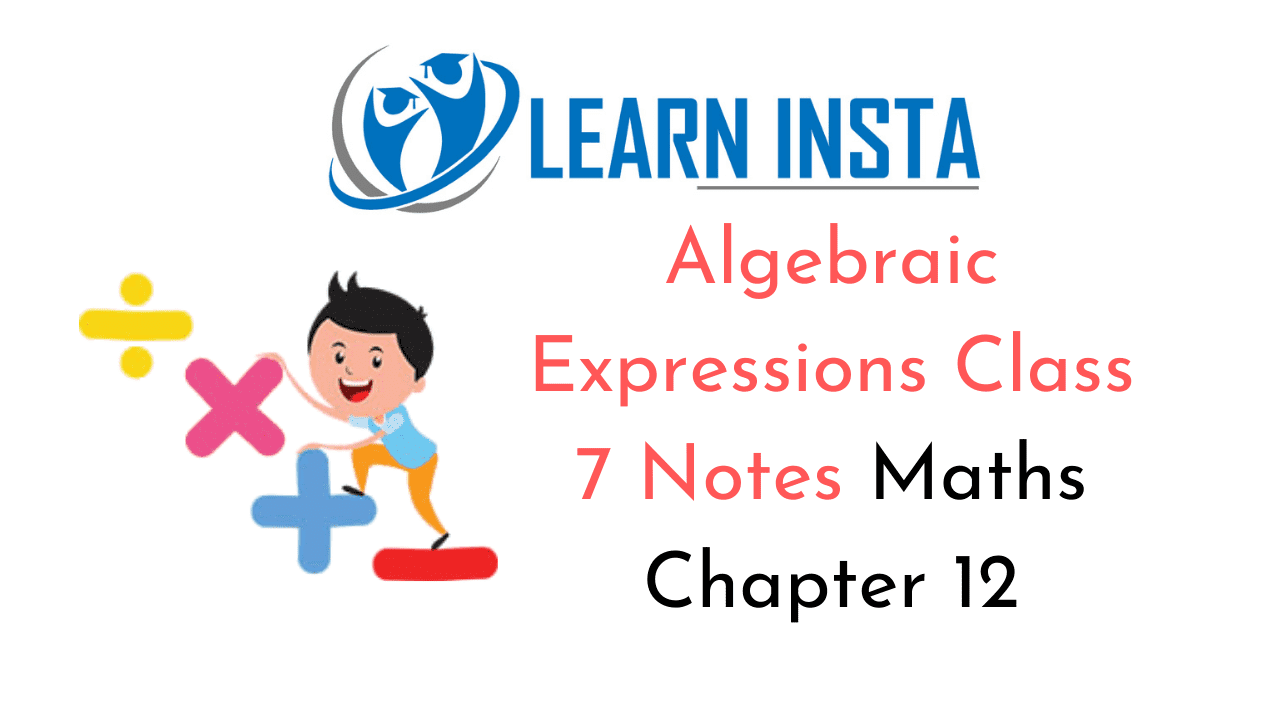On this page, you will find Algebraic Expressions Class 7 Notes Maths Chapter 12 Pdf free download. CBSE NCERT Class 7 Maths Notes Chapter 12 Algebraic Expressions will seemingly help them to revise the important concepts in less time.

## CBSE Class 7 Maths Chapter 12 Notes Algebraic Expressions

### Algebraic Expressions Class 7 Notes Conceptual Facts

1. Algebraic expressions are formed by using variables and constants.
For example: the expression 2x + 3y + 1 is formed by the variables x and y the constants 2, 3 and 1.

2. Expressions are made up terms.
For example: we get an expression if the terms 3xy + 2z, and 3 are added, i.e. 3xy + 22 + 3.

3. Coefficient is the numerical factor in a terms.
For example: In the term 5xy, 5 is the coefficient of xy.

4. Algebraic expressions obey the rules of operations of addition, subtraction, multiplication and division.

5. Like and unlike terms: The terms which have same variable factors are called like terms and the terms which have different variable factors are called unlike terms.
For example:

• 6ab, $$\frac{3}{2}$$ ab, 5ab, – ab are all like terms.
• 3x2y, 5xy, $$\frac{3}{2}$$xy2 are all unlike terms.

Type of Algebraic expressions:

(i) Monomial: An algebraic expression having only one term is called monomial.
For example: 3x, 5y, 6xy, 3x2 are all monomials.

(ii) Binomial: An algebraic expression having two terms is called binomial.
For example: x + y, 3x – y2 , 6x + 5 are all binomials.

(iii) Trinomials: An algebraic expression having three terms is called trinomial.
For example: a2 + b2 + c2 , 2x + 3y – 5, 4x2 + x + y2 are all trinomials.

(iv) Polynomials: An algebraic expression having one or more terms is called polynomial.
For example: a + bx + cx2 + dx3 + … is called a polynomial.